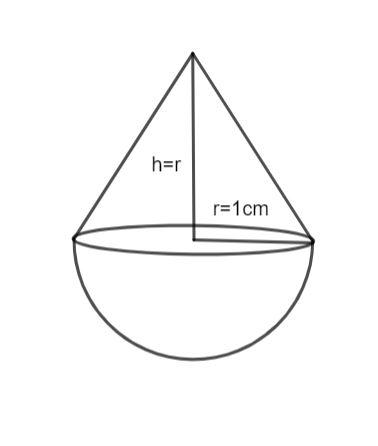QuestionAnswers

# A solid is in the shape of a cone standing on a hemisphere with both their radii being equal to 1 cm and the height of the cone is equal to its radius. Find the volume of the solid in terms of $\pi$.

Hint: The volume of a hemisphere with radius r is $\dfrac{2}{3}\pi {r^3}$ and the volume of the cone with radius r and height h is $\dfrac{1}{3}\pi {r^2}h$. Find the volumes of hemisphere and cone separately and add them to get the required answer.

A hemisphere is half of the sphere cut by the plane passing through its center. The volume of the hemisphere is half of that of the sphere.
A hemisphere with radius r has a volume given as follows:
${V_H} = \dfrac{2}{3}\pi {r^3}...........(1)$
A cone is a right angle triangle that is rotated with any one of its perpendicular sides as the axis in 3-dimensional space. The volume of the cone is one-third of the volume of the cylinder.
The volume of a cone of radius r and height h is given as follows:
${V_C} = \dfrac{1}{3}\pi {r^2}h...........(2)$The solid in the given question is composed of a hemisphere and a cone. We can calculate its volume by calculating separately the volumes of cones and hemisphere and adding them.
The volume of the hemisphere of radius 1 cm is given by equation (1) as follows:
${V_H} = \dfrac{2}{3}\pi {(1)^3}$
${V_H} = \dfrac{2}{3}\pi c{m^3}$
The volume of the cone of radius 1 cm and height 1 cm is given by equation (2) as follows:
${V_C} = \dfrac{1}{3}\pi {(1)^2}(1)$
${V_C} = \dfrac{1}{3}\pi c{m^3}$
Adding the two volumes, we have:
$V = {V_H} + {V_C}$
$V = \dfrac{2}{3}\pi + \dfrac{1}{3}\pi$
$V = \pi c{m^3}$
Hence, the volume of the solid is $\pi$ cubic cm.

Note: The question is asked to find the volume of the solid in terms of $\pi$, hence, leave the answer in terms of $\pi$, if you evaluate, then the answer will be wrong.Students should remember formulas of volume of hemisphere and cone for solving these type of problems.